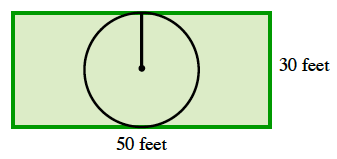### Home > CCA2 > Chapter 2 > Lesson 2.2.1 > Problem2-88

2-88.

Lilia wants to have a circular pool put in her backyard. She wants the rest of the yard to be paved with concrete.

1. If her yard is a $50$ ft. by $30$ ft. rectangle, what is the radius of the largest pool that will fit in her yard?

Draw the rectangle.

Fit the largest possible circle into the rectangle (it does not have to be in the center).

The radius of the pool is $15$ ft.2. If the concrete is to be $8$ inches thick, and costs $\2.39$ per cubic foot, what is the cost of putting in the concrete? No concrete will be used in the pool. (Reminder: Volume $=$ (Base Area $·$ Depth ).

Find the area of the yard (rectangle) and the area of the pool (circle).

Subtract the area of the pool from the area of the yard to find the area that will be paved.

$\text{A}=1500−225\pi\approx793.14\text{ ft.}^2$

$\text{Since 8 inches is } \frac{2}{3}\ \text{of a foot, multiply the area of the region to be paved by two thirds.}$

$V = 793.14 \cdot \frac{2}{3} \approx 528.76 \text{ ft.}^{3}$

Now multiply by the price per cubic foot to find the total cost.

$C=528.76 \text{ ft.}^{3} \cdot 2.39 \frac{\text{dollars}}{\text{ft.}^{3}}$

The total cost is $\1,263.74$.